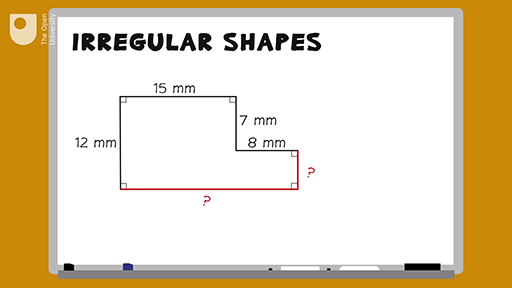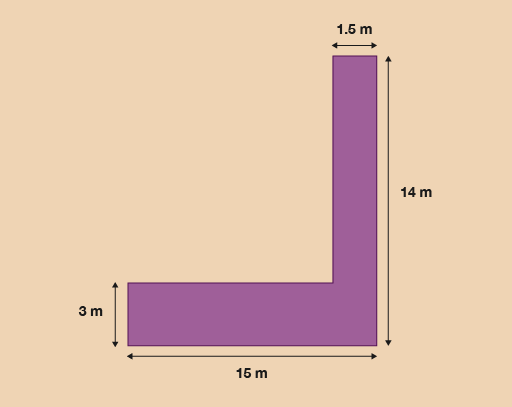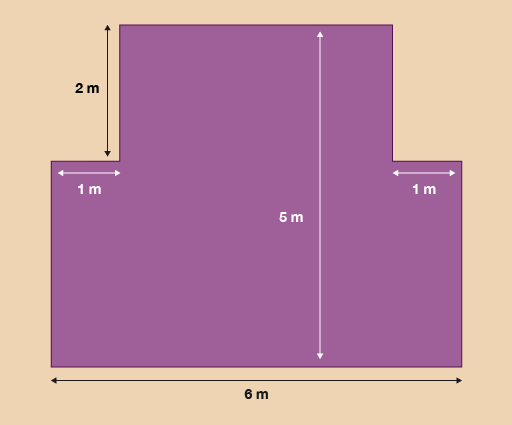Everyday maths 1 (Wales)

Start this free course now. Just create an account and sign in. Enrol and complete the course for a free statement of participation or digital badge if available.

Free course

# 3.1 Measuring the perimeter of irregular shapes

## Example: How to measure the perimeter of an irregular shape

How would you measure the perimeter of an irregular shape – an L-shaped room, for instance – if you didn’t have all of the measurements that you would need? Watch the following video to find out.

Download this video clip.Video player: 19_ani_irregular_shapes.mp4Skip transcript

#### Transcript

Say you needed to find the perimeter of this shape, but two of the measurements are missing. How would you find them out? You can assume that all of the corners are right angles. You need to use the measurements that you do know.

So if you want to find out the missing side on the right, you know that the left-hand side opposite to this is 12 millimetres long. And there is another measurement on the same side in the middle of the shape that is 7 millimetres long.

The missing length must then be the difference between 7 and 12 millimetres. Here’s a sum to show this: 12 minus 7 equals 5 millimetres. So the answer for the right-hand side is 5 millimetres. You can check this by adding 5 and 7, which makes 12 millimetres, the same as the left-hand side.

To find out the length at the bottom of the shape, because you know that all of the corners are right angles, you can add together the other two parallel measurements that are given: 15 plus 8 equals 23 millimetres. So the bottom of the shape is 23 millimetres.

Now you know the measurements for all of the shape’s edges, you can measure the perimeter. 12 plus 15 plus 7 plus 8 plus 5 plus 23 equals 70 millimetres. So the perimeter is 70 millimetres.

End transcript

Interactive feature not available in single page view (see it in standard view).

Now try the following activity. Remember to check your answers once you have completed the questions.

## Activity 8: Finding the perimeter

Note that you can assume that all of the corners in the images in this activity are right angles.

1. A gardener decides to lay a new path next to his lily pond. The drawing shows the dimensions of the path.Figure 18 A pathway

The gardener decides to paint a white line around the perimeter of the path. What is the perimeter of the path?

2. A tourist information centre has a new extension.Figure 19 A new extension

The tourist board wants to attach a gold strip around the border of the floor of the building. What is the perimeter of the new extension?

### Answer

1. To calculate the missing sides you should have carried out the following calculations:

• 14 – 3 = 11
• 15 – 1.5 = 13.5

Now that you have found the missing sides, you can add them all together:

• 15 + 14 + 1.5 + 3 + 13.5 + 11= 58 m
2. To calculate the missing sides you need to carry out the following calculations to calculate the perimeter:

• 5 – 2 = 3
• 6 – 2 = 4

Now that you have found the missing sides, you can add them all up together to calculate the perimeter:

• 6 + 3 + 3 + 1 + 1 + 2 + 2 + 4 = 22 m

## Summary

In this section you have learned how to work out the perimeter of both simple and irregular shapes.

FSM_1_CYMRU

### Take your learning further

Making the decision to study can be a big step, which is why you'll want a trusted University. The Open University has 50 years’ experience delivering flexible learning and 170,000 students are studying with us right now. Take a look at all Open University courses.

If you are new to University-level study, we offer two introductory routes to our qualifications. You could either choose to start with an Access module, or a module which allows you to count your previous learning towards an Open University qualification. Read our guide on Where to take your learning next for more information.

Not ready for formal University study? Then browse over 1000 free courses on OpenLearn and sign up to our newsletter to hear about new free courses as they are released.

Every year, thousands of students decide to study with The Open University. With over 120 qualifications, we’ve got the right course for you.

Request an Open University prospectus371